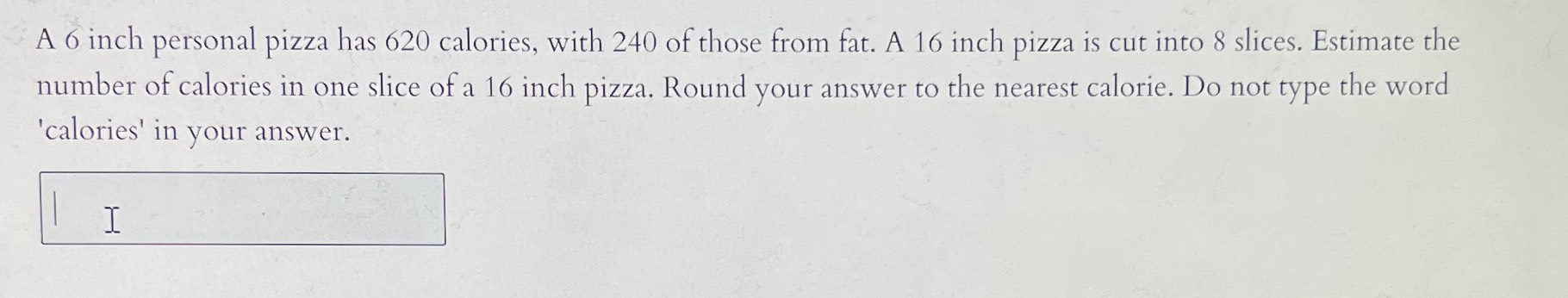### ¿Todavía tienes preguntas de matemáticas?

Pregunte a nuestros tutores expertos
Algebra
Pregunta$$6$$ inch personal pizza has $$620$$ calories, with $$240$$ of those from fat. A $$16$$ inch pizza is cut into $$8$$ slices. Estimate the number of calories in one slice of a $$16$$ inch pizza. Round your answer to the nearest calorie. Do not type the word 'calories' in your answer.

$$\frac{6}{620}= \frac{16}{x},x= 1653.33$$